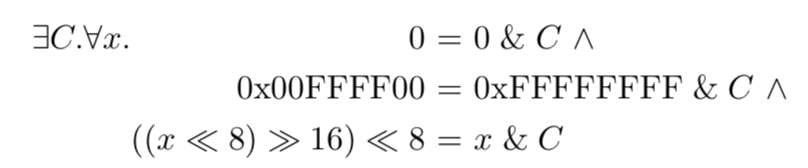regehr.org Go back Open original

# Synthesizing Constants

By regehr

(See this blog post for a short introduction to synthesis, or this paper for a long one.)

In this piece I want to discuss an aspect of program synthesis that sounds like it should be easy, but isn’t: synthesizing constant values. For example, consider trying to synthesize an optimized x86-64 implementation of this code:

` long foo(long x) { return x / 52223; } `

The desired output is something like:

` foo(long): movabs rdx, -6872094784941870951 mov rax, rdi imul rdx lea rax, [rdx+rdi] sar rdi, 63 sar rax, 15 sub rax, rdi ret `

Assume for a moment that we know that this sequence of instructions will work, and we only lack the specific constants:

` foo(long): movabs rdx, C1 mov rax, rdi imul rdx lea rax, [rdx+rdi] sar rdi, C2 sar rax, C3 sub rax, rdi ret `

We need to find a 64-bit constant and two 6-bit constants such that the assembly code returns the same result as the C code for every value of the function parameter. Of course there’s a well-known algorithm that GCC and LLVM use to compute these constants, but the synthesizer doesn’t have it: it must operate from first principles.

An even more difficult example is a 64-bit Hamming weight computation, where (assuming we don’t want to use vector instructions) we might hope to synthesize something like this:

` popcount64(unsigned long): movabs rdx, 6148914691236517205 mov rax, rdi shr rax and rax, rdx movabs rdx, 3689348814741910323 sub rdi, rax mov rax, rdi shr rdi, 2 and rax, rdx and rdi, rdx add rdi, rax mov rax, rdi shr rax, 4 add rax, rdi movabs rdi, 1085102592571150095 and rax, rdi movabs rdi, 72340172838076673 imul rax, rdi shr rax, 56 ret `

Again assuming that we know that this sequence of instructions will work, we still need to come up with 280 bits worth of constant values. One can imagine even more challenging problems where we want to synthesize an entire lookup table.

The running example I’ll use in this post is much easier:

` uint32_t bar1(uint32_t x) { return ((x << 8) >> 16) << 8; } `

The code we want to synthesize is:

` uint32_t bar2(uint32_t x) { return x & 0xffff00; } `

That is, we want to find C in this skeleton:

` uint32_t bar3(uint32_t x) { return x & C; } `

## Basic Constant Synthesis

We need to solve an “exists-forall” problem: does there exist a C such that the LHS (left-hand side, the original code) is equivalent to the RHS (right-hand side, the optimized code) for all values of x? In other words:(Here we’ll play fast and loose with the fact that in the real world we check refinement instead of equivalence — this doesn’t matter for purposes of this post.)

The specific formula that we want an answer for is:A SAT solver can natively solve either an exists query (this is the definition of SAT) or else a forall query (by seeing if there exists a solution to the negated proposition). But, by itself, a SAT solver cannot solve an exists-forall query in one go. An SMT solver, on the other hand, can natively attack an exists-forall query, but in practice we tend to get better results by doing our own quantifier elimination based only on SAT calls. First, we ask the solver if there exists a C and x that make the LHS and RHS equivalent. If not, synthesis fails. If so, we issue a second query to see if C works for all values of x. If so, synthesis succeeds. If not, we add a constraint that this value of C doesn’t work, and we start the process again. The problem is that each pair of queries only rules out a single choice for C, making this process equivalent, in the worst case, to exhaustive guessing, which we cannot do when C is wide. We need to do better.

## Reducing the Number of Counterexamples using Specialization

Souper uses a technique that appears to be common knowledge among people who do this kind of work; unfortunately I don’t know where it was first published. The trick is simple: we choose a few values of x and use it to specialize both the LHS and RHS of the equation; you can think of this as borrowing a few constraints from the forall phase and pushing them into the exists phase. In many cases this adds enough extra constraints that the solver can arrive at a workable constant within a few iterations. If we specialize x with 0 and -1 then in the general case we get:After constant folding, our running example becomes:The first choice, 0, turns out to be an unlucky one: after further simplification it comes out to “0 = 0”, giving the solver no extra information. The second choice, on the other hand, is extremely lucky: it can be rewritten as “0x00FFFF00 = C” which simply hands us the answer. In most cases things won’t work out so nicely, but specializing the LHS and RHS with fixed inputs helps enormously in practice.